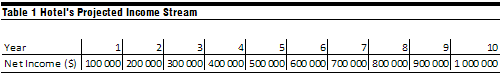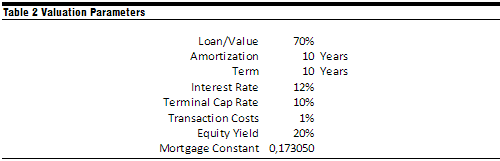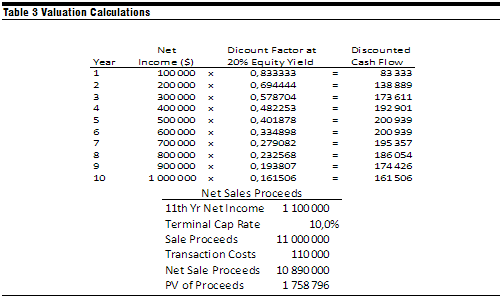## Hotel Valuation Techniques

Hotel appraising is done in different markets across the world and valuation experts generally consider three approaches to valuing a hotel asset. What are the pros and cons of each approach and which is more applicable when valuing your property?

In this article we will provide an overview of lodging valuation approaches. Appraisers use three approaches when deriving a property’s Market Value1:

• Income Capitalization Approach
• Sales Comparison Approach
• Cost Approach

Although all three valuation approaches are generally given consideration, the inherent strengths of each approach and the nature of the hotel in question must be evaluated to determine which approach will provide supportable value estimates.

Income Capitalization Approach

The income capitalization approach is based on the principle that the value of a property is indicated by its net return, or what is known as the "present worth of future benefits." The future benefits of income-producing properties, such as hotels, are the net income, estimated by a forecast of income and expense, along with the anticipated proceeds from a future sale. These benefits can be converted into an indication of market value through a capitalization process and discounted cash flow analysis.

We consider a ten-year leveraged discounted cash flow to be the most accurate method of valuation, provided there is a transparency for the appraiser to prove the source of all market assumptions and investment parameters. Once the appraiser has forecasted all future income and expenses for the property, the hotel’s future earnings can be estimated. The appraiser then assumes an optimum capitalization rate, which reflects the risk of owning a hotel over that period. We further assume the sale of the asset at the end of the ten-year period. We then discount the forecasted earnings and the sale proceeds to the present value based on the investment parameters and return requirements of equity and debt participants.

This approach is generally considered to be the preferred method of valuation for income-producing properties, because it most closely reflects the investment thinking of knowledgeable buyers.

Sales Comparison Approach

The sales comparison approach is based on the assumption that an informed buyer will pay no more for a property than the cost of acquiring an existing property with similar utility. To obtain a supportable value, the sales proceeds from the similar property should be adjusted to reflect the differences between the two assets. While hotel investors are interested in the information contained in the sales comparison approach, they usually do not employ this approach in reaching their final purchase decisions. Factors such as the lack of recent sales data and the numerous insupportable adjustments that are necessary often make the results of this technique questionable.

The sales comparison approach is most useful in providing a range of values indicated by prior sales and in establishing an indicator of pricing momentum; however, reliance on this method beyond the establishment of broad parameters is rarely justified by the quality of the sales data.

Cost Approach

The cost approach is based on the assumption that an informed buyer will pay no more for a property than the cost of building a brand new property with similar utility. The value is obtained by calculating the current cost of replacement and subtracting any depreciation factors, such as physical deterioration, and functional and economic obsolescence. The depreciated amount should than be added to the value of land, as though it were vacant and available to reach an estimated total value. The cost approach may provide a reliable estimate of value in the case of new properties, but as buildings and other improvements grow older and begin to deteriorate, the resultant loss in value becomes increasingly difficult to quantify accurately. This method is however useful in determining the cost to enter the marketplace or when valuing newer properties.

We find that most knowledgeable hotel buyers base their purchase decisions on economic factors such as projected net income and return on investment. Because the cost approach does not reflect these income-related considerations and requires a number of highly subjective depreciation estimates, this approach is given minimal weight in the hotel valuation process.

Conclusion

The above valuation methods are globally recognized as the main approaches to valuing hotel assets. Even though the most relevant of these is the income capitalization approach, the prudent appraiser will consider all three methods. The cost approach sometimes indicates the “cost of entry” into a particular market, whereas the sales comparison approach would normally provide a range of values on the per room basis.

Valuation example using Income Capitalization Approach

As mentioned earlier in this article, the income capitalization approach is generally the preferred valuation method for income producing properties. Let us take a look at the following example of how to derive an asset’s value using the income capitalization approach. Table 1 below represents an asset’s income stream.Also as mentioned earlier, in order to derive an asset’s value using the income capitalization approach we will require to compute the present value of future income streams along with the sales proceeds from the sale of the asset. Table 2 below represents the valuation parameters used in this example.Since we know that debt will be required to purchase this asset, we can deduce that the value of this property will be the sum of the debt and equity components:

Total Asset Value (V) = Value of Equity (E) + Value of Debt (D)

E = (1-M)V
where M = Loan to Value Ratio

If we can calculate the Value of Equity for the asset, we will be able to calculate the Total Asset Value. We know that the Value of Equity is the sum of the present values of annual net incomes to equity and the present value of cash flow from the sale of the asset.

(1-M)V = ∑ (De x 1/Sn) + ((NI11/Rr) – [b(NI11/Rr)])x1/S10
Where De = NI – (f x M x V)

 NI = Net income available for debt service V = Value M = Loan-to-value ratio f = Mortgage Constant De = Annual cash available to equity b = Transaction Costs n = Number of years in the projection period Rr = Terminal capitalization rate

1/Sn = Present worth of \$1 factor (discount factor) at the equity yield rate

Table 3 below represents all the calculations required to fulfill the above equation.Having all the above data we can rewrite the equation and simplify to get the following:

(1-M)V = 1 707 955 – 0,507854 V + 1 758 796

0,807854 V = 3 466 751

V = 4 291 309

We have just shown the value of the asset with the projected income streams from Table 1 and the valuations parameters from Table 2.

It is important to remember that the above example uses hypothetical numbers and a very simplified valuation scenario; however, the formula and methodology of the income capitalization approach will apply to any actual asset. The main challenge behind a skilled valuation is deriving all the necessary data required for valuation of hospitality assets and should be trusted to professional hospitality consultants and appraisers.

1 Definition of Market Value: ‘the estimated amount for which an asset should exchange on the date of valuation between a willing buyer and a willing seller in an arm’s length transaction after proper marketing wherein the parties had each acted knowledgably, prudently and without compulsion.’
-RICS (2008) Appraisal and Valuation Standards

1.Ethan ChangMarch 14, 2011

Hello Alexey, We are a lender of Strategic Hotel. And we know this Hotel Valuation Approach has been used to appraise the value of Stategic Hotel. We want to know if the Hotel Valuation Approach is already used to any other properties. And is this approach now acceptable by the market now? Han Commercial Bank, Los Angeles Branch Ethan Chang 張光儀 707 Wilshire Blvd., Suite 3100, Los Angeles, CA90017 Tel : 213-362-6666 ext. 234 Fax: 213-362-6617 E-mail: [email protected]

2.Alexey, this article took a great deal of time and research. Since valuation techniques are our bread and butter, I think this will bring you a lot of exposure and result in potential client calls and business. I am a great proponent of writing articles and industry appearances, and want to congratulate you for your efforts. Steve

3.jeyan akcayMarch 21, 2011

Hi, Alex, I read your article with great interest; very useful indeed since it is debt involved. Just one question to complete my understanding: If the total value (M) is 4 291 309 m \$, should I then assume that the balance between M and 0,80M (824,558 \$) goes to equity? Any contribution would be helpful. Thanks.